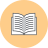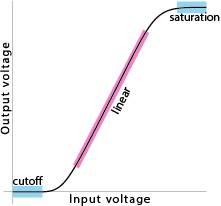## Bits

In this lab, you will explore how different kinds of information are represented in a computer.

: Bit
DAT-1.A.3

A bit is a single unit of data that can only have one of two values. We usually represent the two values as 0 (off) and 1 (on).

As you probably know, information travels over wires inside the computer, and each wire is either on or off, with no intermediate states allowed. This small piece of information is called a bit, the smallest possible unit of information in the digital domain.

What does the value of a bit mean? By convention, the two states of a bit are interpreted as 0 and 1, but that doesn't mean they have to represent numbers. A single bit can represent

• False and True
• Off and On, simulating a light switch
• Red and Green, simulating a traffic light
• ...and many more

But what if the traffic light also needs a yellow value? It's tempting to say that, for example, 0 volts on the wire means red, 1 volt means yellow, and 2 volts means green. Long ago, there were computers that worked that way, but there are good reasons to stick with two possible values per wire.What good reasons?The fundamental building block of computer circuitry is the transistor. In a digital computer, the input to a transistor is either zero or whatever voltage represents one. But electrical circuits aren't perfect; the input may be a little larger or smaller than it should be.

This is a rough graph of the actual input-output behavior of a transistor. Don't worry about the details; just notice the two blue flat parts of the graph. Within the "cutoff" region, small changes to the input voltage do not change the output voltage at all; the output is always zero volts. Likewise within the "saturation" region, small input changes don't affect the output voltage; this output is interpreted as a one. This is how transistors are used as switches in a computer. If there were three flat parts of the curve, maybe we would have three possible values for each wire.

Transistors are versatile devices. When used in the middle, linear (pink) part of the graph, they're amplifiers; a small variation in input voltage produces a large variation in output voltage. That's how they're used to play music in a stereo.

DAT-1.A.2
Instead of multiple-valued wires, we use more than one bit if we need to represent more than two possible values. So, for the traffic light, we could use two bits:
first bit second bit meaning
0  red
1  yellow
0  green
1    (unused)

There are four possible combinations of two bits, so with two bits we can represent up to four different values, even though we only need three for the traffic light.

1. Convince yourself that there aren't any more combinations of two bits.
2. Write down all the possible combinations of three bits. How many are there?
3. How many combinations of four bits are there?

Each added bit doubles the number of values you can represent. This means that representing complex situations doesn't cost a lot of hardware; ten bits is enough to represent over 1000 distinct values.

1. How many values, exactly, can be represented by ten bits?
2. How many bits do you need to represent the days of the week?
3. How many bits do you need to represent one decimal digit (that is, to specify a digit 0-9)?
: Byte and Word
DAT-1.A.4

A byte is eight bits.

A word is a sequence of however many bits the CPU processes at a time. As of 2017, words are 32 or 64 bits.

Bits aren't expensive, but what is expensive is the circuitry to let the programmer use exactly the smallest number of bits for a particular problem.

Can we cut this commented out text? --MF, 8/31/19
I think we should consider moving it to U6, but not tonight... -bh
Instead, modern computers generally allow memory allocation in only two sizes: the byte, which is standardized at eight bits, and the word, which is defined as a sequence of however many bits the CPU processes at a time. As of 2017, words can be 32 bits or 64 bits wide, although most new computer models use 64 bits.

The exact answer for 32 bits is 4,294,967,296 so this approximation is pretty close.

How many distinct values can be represented in 32 bits? You don't have to memorize the answer, because you can quickly approximate it using the fact that 210 = 1024, which is about 1000. This means that every ten bits of width multiplies the number of values that can be represented by about 1000. So, 10 bits allows about a thousand values, 20 bits is about a million values, 30 bits is about a billion, and 32 bits allows over four billion values (because we double the billion two more times for the difference between 30 and 32).

You might find this trick helpful on the AP exam.

Four billion values sounds like it ought to be enough, but it's not if you're an astronomer or a banker (or Google or Facebook). That's why we now have 64-bit computers, which as of 2019 are the standard. (Apple has just removed support for 32-bit programs from MacOS.)

1. About how many different values can be represented in a 64-bit word? (Don't use a calculator; use the trick!)

### Bytes and Characters

The main use of eight-bit bytes is to represent characters of text.

1. How many bits do you need to represent the 26 letters in English and the ten digits 0-9?
The widespread use of eight-bit ASCII is the main historical reason why the eight-bit byte became standard. (Another reason is that computer circuitry can most easily deal with widths that are powers of two.)

Computers used six-bit-wide character codes for many years, but to have both UPPER CASE and lower case letters and punctuation requires seven bits. The first officially recognized character encoding was the seven bit ASCII (American Standard Code for Information Interchange) character set. It included an optional eighth bit for error detection, which was taken over to include accented characters in Spanish, French, German, and some other European languages. For example, there is an accented character in the name of the main developer of Snap!, Jens Mönig, who is German. (The closest English sound is the "u" in "lunch.")

As the use of computers and the Internet spread around the world, people wanted to be able to write Chinese, Japanese, Arabic, Kabyle, Russian, Tamil, etc. The Unicode character set supports about 1900 languages, using 32 modern alphabets and 107 historical alphabets that are no longer in living use. The complete Unicode character set includes 136,755 characters.

1. What's the minimum number of bits needed to represent any Unicode character?The actual computer representation of Unicode is complicated.

The most straightforward representation of Unicode uses one 32-bit word per character, which is more than enough. But program developers consider that an inefficient use of computer memory, and also, a lot of old software still in use was written when eight bits per character was standard. So Unicode characters are generally represented in a multi-byte representation in which the original 128 ASCII characters occupy one byte, while other characters may require up to four bytes. (It's also possible to use a multi-byte sequence to tell your word processing software that you want to use one-byte or two-byte codes to represent a particular non-Latin alphabet.)

1. This question is similar to those you will see on the AP CSP exam.
Which of the following CANNOT be expressed using one bit?
The state of an ON/OFF switch
The value of a Boolean variable
The remainder when dividing a positive integer by 2
The position of the hour hand of a clock